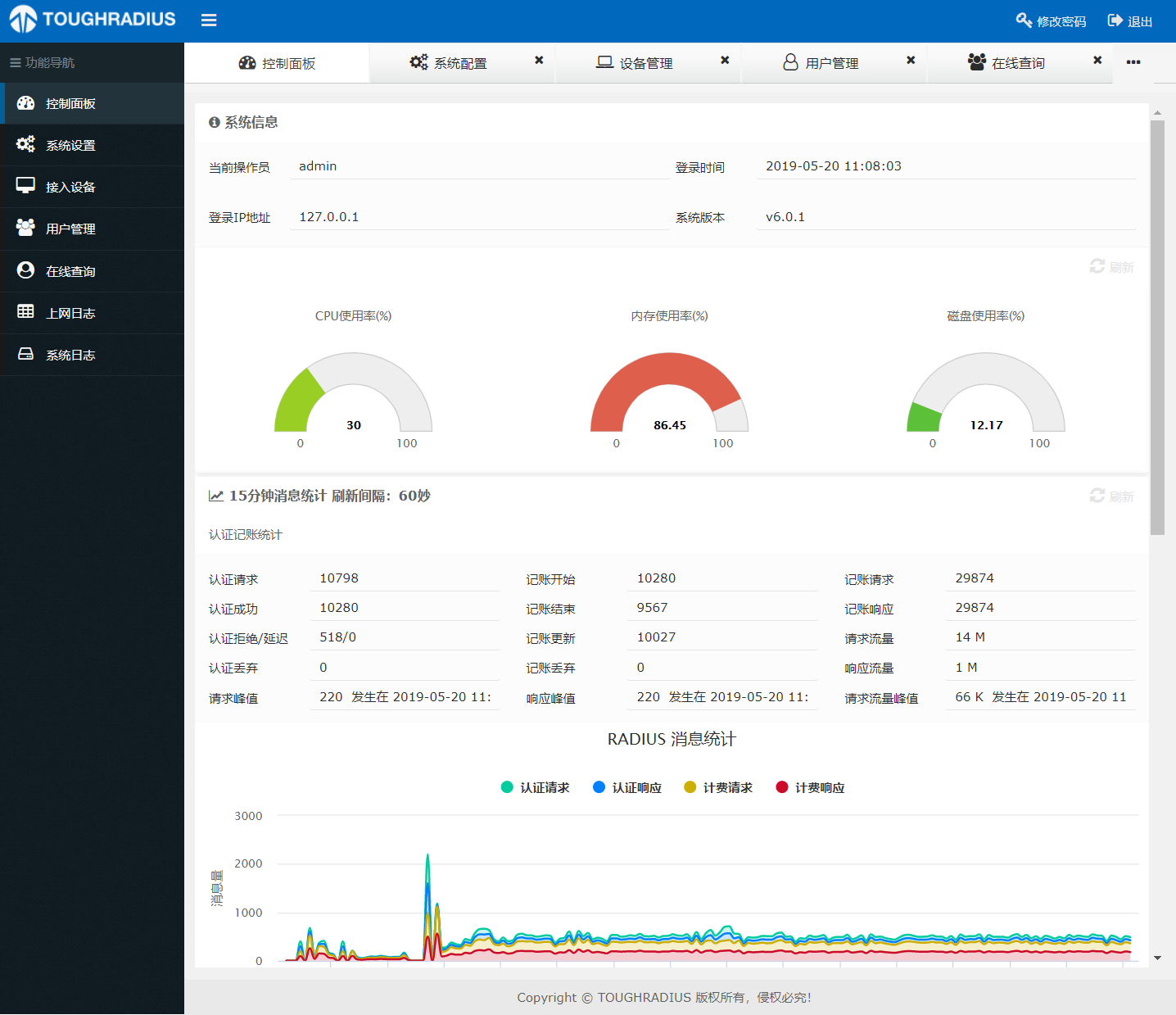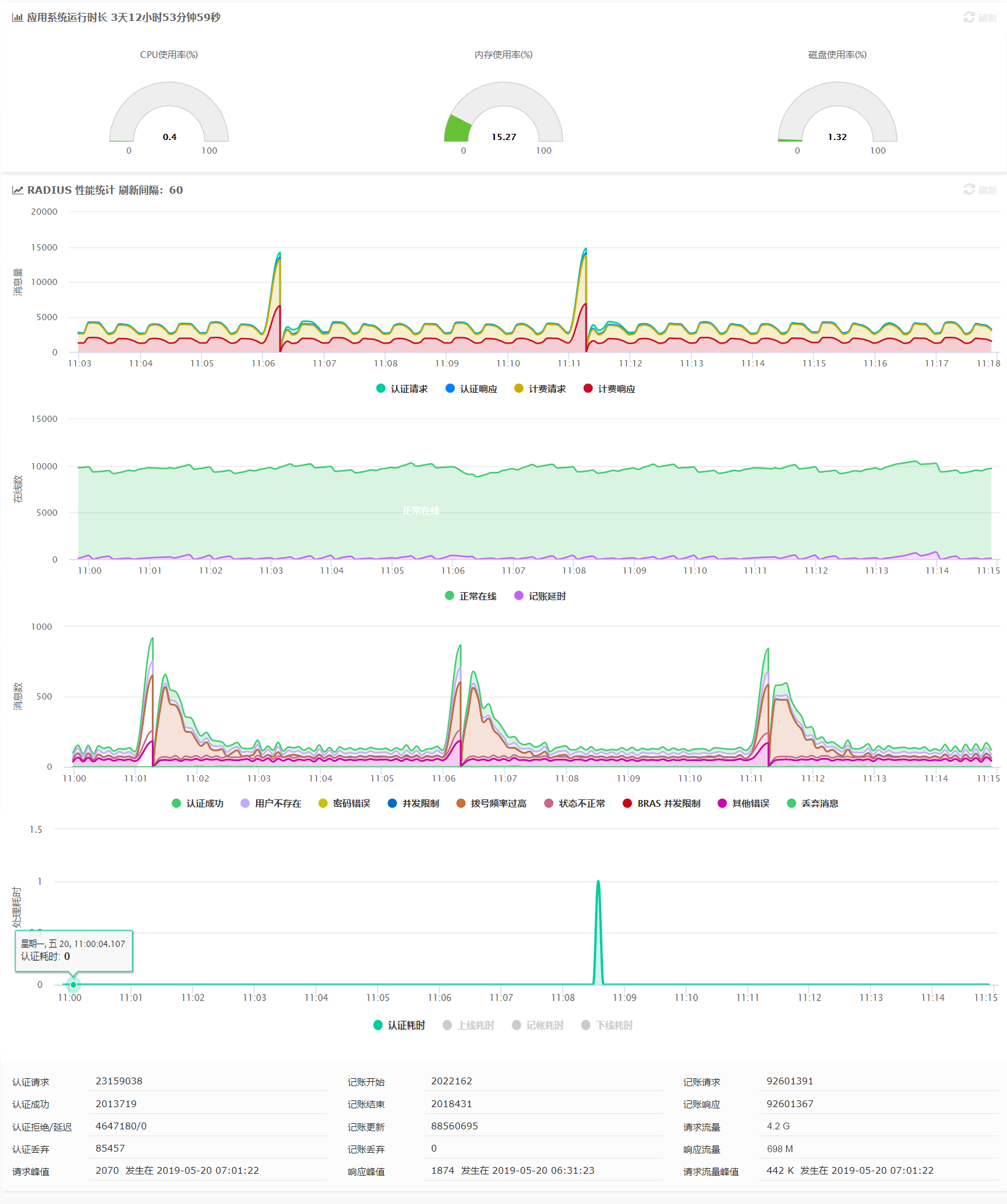CIIS 2019丨CIIS 2019丨倒计时10天丨CIIS 2019 嘉宾阵容公布！>>>)                        )   (                (       (              (
*   )   ( /(            (        ( /(   )\ )     (       )\ )    )\ )           )\ )              (
` )  /(   )\())      (    )\ )     )\()) (()/(     )\     (()/(   (()/(      (   (()/(     (   (    )\ )
( )(_)) ((_)\       )\  (()/(    ((_)\   /(_)) ((((_)(    /(_))   /(_))     )\   /(_))    )\  )\  (()/(
(_(_())    ((_)   _ ((_)  /(_))_   _((_) (_))    )\ _ )\  (_))_   (_))    _ ((_) (_))     ((_)((_)  /(_))
|_   _|   / _ \  | | | | (_)) __| | || | | _ \   (_)_\(_)  |   \  |_ _|  | | | | / __|    \ \ / /  (_) /
| |    | (_) | | |_| |   | (_ | | __ | |   /    / _ \    | |) |  | |   | |_| | \__ \     \ V /    / _ \
|_|     \___/   \___/     \___| |_||_| |_|_\   /_/ \_\   |___/  |___|   \___/  |___/      \_/     \___/

/)
_   __   __   _    _/_  ___       _  (/   _  _/_  __      _ _/_      _  ______
(_(/ (_(/ (_(/  .  (__ (_) (_(_  (_/_/ )_/_)_(__ / (_(_(_(__(__  .  (__(_) // (_
.-/
(_/

TOUGHRADIUS V6 是一个全新设计的版本，是的，在V5的时候我也这么说过，但是这次真的不一样的，因为 V6 已经被真正的用在了生产环境中。

V6 版本采用 java 重新开发，但真正重大意义不在于开发语言，而是V6版本更贴近用户，比如更易于部署，更易于开发扩展，更关注使用体验，TOUGHRADIUS V1，V2版本参杂太多的业务而变得复杂，V3，V4 版本是没有出现过的版本， V5是一次失败的尝试，而 V6 是重生。技术实现

RADIUS ( Remote Authentication Dial In User Service 远程认证拨号用户服务 )是一种在网络接入设备和认证服务器之间承载认证、授权、计费（AAA）和配置信息的协议。RADIUS 协议是在认证、授权、计费方面应用最为广泛的协议之一具有以下特点：

• 客户端/服务器结构
• 采用共享密钥保证网络传输安全性
• 良好的可扩展性
• 认证机制灵活

Portal技术

Portal在英语中是入口的意思。Portal认证通常也称为Web认证，一般将Portal认证网站称为门户网站。

Portal认证技术则提供一种灵活的访问控制方式，不需要安装客户端，就可以在接入层以及需要保护的关键数据入口处实施访问控制。